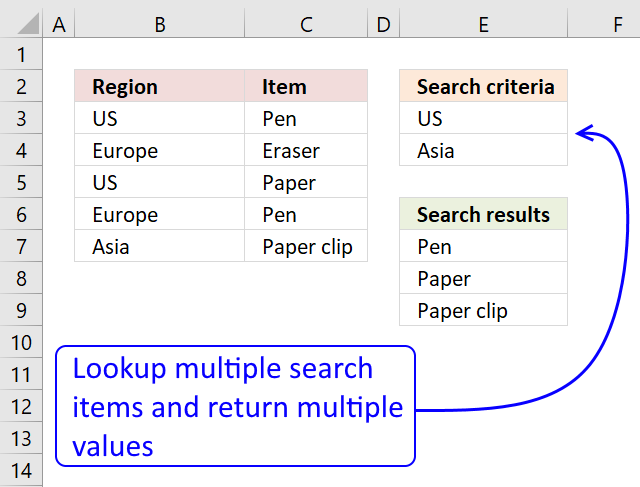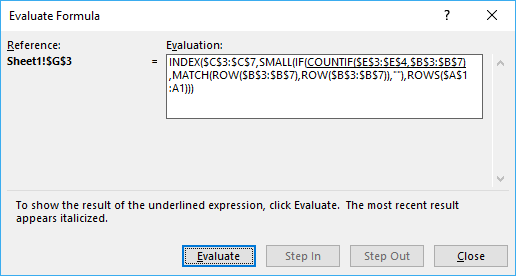Author: Oscar Cronquist Article last updated on December 18, 2020

In this article I'll show you how to lookup two or more values in a list and return (if possible) multiple matches.

The picture below shows you a table in column B and C, the search criteria is in column B and the results are in column G.I am not using VLOOKUP at all in this array formula, the VLOOKUP looks for a value in the leftmost column of a table, and then returns a value in the same row from a column you specify.

The VLOOKUP function is not designed to look for multiple values and return multiple values.

Update 18 December 2020, the new FILTER function is now available for Excel 365 users, formula in cell E7:

=FILTER(\$C\$3:\$C\$7, COUNTIF(\$E\$3:\$E\$4, \$B\$3:\$B\$7))

This formula is entered as a regular formula, read here how the formula works in detail: Filter values based on criteria

The formula below is for earlier Excel versions, array formula in E7:

=INDEX(\$C\$3:\$C\$7, SMALL(IF(COUNTIF(\$E\$3:\$E\$4, \$B\$3:\$B\$7), MATCH(ROW(\$B\$3:\$B\$7), ROW(\$B\$3:\$B\$7)), ""), ROWS(\$A\$1:A1)))

Recommended article

Get the smallest number larger than a given number

This article demonstrates how to extract the smallest number larger than a condition and the largest number smaller than a […]

### How to enter an array formula

1. Copy the aray formula above (Ctrl + c)
2. Double press with left mouse button on cell G3
3. Paste (Ctrl + v)
4. Press and hold Ctrl + Shift simultaneously
5. Press Enter
6. Release all keys

If you made the above steps correctly the formula now has a beginning and ending curly bracket, like this:
{=array_formula)}

Don't enter these characters yourself, they appear automatically.

A beginners guide to Excel array formulas

Array formulas allows you to do advanced calculations not possible with regular formulas.

### How to copy the formula to cells below

1. Select cell E7
2. Copy cell (Ctrl + c)
3. Select cell range E8:E9
4. Paste (Ctrl + v)

### How the array formula works in cell E7

You can easily follow a long as I explain the array formula, get the workbook. Select cell B13, go to tab "Formulas". Press with mouse on "Evaluate formula" button.Press with mouse on "Evaluate" button show above to move to next step.

#### Step 1 - Count matching search criteria in column B

COUNTIF(\$E\$3:\$E\$4, \$B\$3:\$B\$7)

becomes

COUNTIF({"US";"Asia"},{"US";"Europe";"US";"Europe";"Asia"})

and returns {1;0;1;0;1}

How to use the COUNTIF function

Counts the number of cells that meet a specific condition.

#### Step 2 - Convert boolean array into corresponding row numbers

IF(COUNTIF(\$E\$3:\$E\$4, \$B\$3:\$B\$7), MATCH(ROW(\$B\$3:\$B\$7), ROW(\$B\$3:\$B\$7)), "")

becomes

IF({1;0;1;0;1}, MATCH(ROW(\$B\$3:\$B\$7), ROW(\$B\$3:\$B\$7)), "")

becomes

IF({1;0;1;0;1}, {1;2;3;4;5}, "")

and returns {1;"";3;"";5}

These are the row numbers that correspond to the matching values US and Asia in column B.

How to use the IF function

Checks if a logical expression is met. Returns a specific value if TRUE and another specific value if FALSE.

#### Step 3 - Returns the k-th smallest row number

SMALL(IF(COUNTIF(\$E\$3:\$E\$4,\$B\$3:\$B\$7), MATCH(ROW(\$B\$3:\$B\$7), ROW(\$B\$3:\$B\$7)), ""),ROWS(\$A\$1:A1))

becomes

SMALL({1;"";3;"";5}, ROWS(\$A\$1:A1))

becomes

SMALL({1;"";3;"";5}, 1)

and returns 1.

How to use the SMALL function

The SMALL function lets you extract a number in a cell range based on how small it is compared to the other numbers in the group.

#### Step 4 - Return a value based on coordinate

INDEX(\$C\$3:\$C\$7, SMALL(IF(COUNTIF(\$E\$3:\$E\$4, \$B\$3:\$B\$7), MATCH(ROW(\$B\$3:\$B\$7), ROW(\$B\$3:\$B\$7)), ""), ROWS(\$A\$1:A1)))

becomes

INDEX(\$C\$3:\$C\$7, 1)

becomes

INDEX({"Pen";"Eraser";"Paper";"Pen";"Paper clip"}, 1)

and returns Pen in cell E7.

How to use the INDEX function

Gets a value in a specific cell range based on a row and column number.

### Get excel *.xlsx file

Vlookup with multiple search conditions and return multiple matches.xlsx